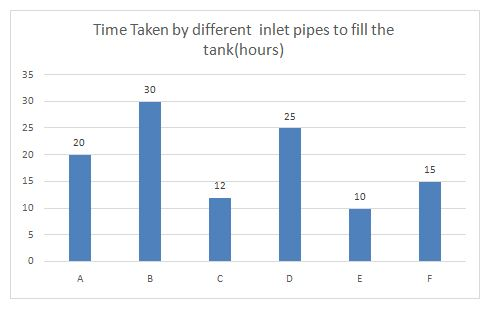# Quantitative Aptitude Questions (Data Interpretation) for SBI PO Mains 2018 Day-153

Dear Readers, SBI is conducting Online Examination for the recruitment of probationary officer. To enrich your preparation here we have providing new series of Data Interpretation – Quantitative Aptitude Questions. Candidates those who are appearing in SBI PO Mains Exams can practice these Quantitative Aptitude average questions daily and make your preparation effective.

Click “Start Quiz” to attend these Questions and view Solutions

Directions (1-5): Study the following information carefully and answer the given questions:The Table below shows time taken by different outlet pipes to empty the tank:

 Pipes Time ( Hours) P 15 Q 10 R 12 S 6 T 8 U 15

1) Pipe A and C were opened simultaneously to fill an empty tank. After some time pipe C was closed, then pipe A took 4 hours to fill the rest of the tank. For how much time was the pipe A and B opened together?

a) 6

b) 18

c) 4

d) 7

e) 2

2) Pipe D and F were opened to fill the empty but when the tank was 64% full Pipe B was also opened find the total time taken to fill the tank?

a) 8(4/7)

b) 2(3/8)

c) 5(2/19)

d) 4(1/9)

e) None of these

3) If Pipes A and B were opened together to fill a tank but accidentally pipe P was also opened  with Pipe A and B then find the time taken to fill the empty tank?

a) 56

b) 24

c) 25

d) 60

e) 14

4) The pipes C and E filled the empty tank of capacity 3300 liters in 5(5/11) hours then find how many liters of water pipe C fills in one hour?

a) 250

b) 370

c) 156

d) 275

e) 450

5) If pipes S and T were used to drain water from the tank but due to hurry these pipes were not opened properly, so the pipes allowed 1/2th and 2/3 part of water respectively, how long will they take to empty the tank?

a) 12

b) 24

c) 6

d) 7

e) None of these

Directions (6-10): Read the following information carefully and answer the following questions carefully. The table shows different kinds of balls in each bag.

 Types of Balls Bag A Bag B Bag C White 2 4 8 Red 4 3 3 Green 6 2 6 Yellow 3 4 5

6) Three balls are drawn at random from Bag A. What is the probability that all the three are of same color?

a) 5/91

b) 9/91

c) 15/91

d) 19/91

e) 14/93

7)  Two balls are picked at random from Bag B. What is the probability that both the balls are white color?

a) 1/13

b) 9/91

c) 15/91

d) 19/91

e) 14/93

8) Three Balls are drawn at random from Bag A. What is the probability that at least two red balls are drawn?

a) 1/13

b) 2/13

c) 4/13

d) 8/13

e) 7/13

9) Three balls are drawn at random from Bag B. What is the probability that at least one ball is of Red color?

a) 42/143

b) 44/143

c) 46/143

d) 83/143

e) None of these

10) Four balls are drawn at random from Bag A. What is the probability that all the balls are of different colors?

a) 42/455

b) 44/455

c) 46/455

d) 48/455

e) None of these

Total units of work= 60

Pipe A’s one hour work= 3 units

Pipe C’s one hour work= 5 units

Work done by pipe A in 4 hours to fill the rest of the tank= 3*4= 12 units

Remaining work was done by pipe A and C= 60-12= 48

Time for which pipe C was open = 48/8= 6 hours

Total units of work= 150

Pipe D’s one hour work= 6 units

Pipe F’s one hour work= 10 units

Pipe B’s one hour work= 5 units

Time taken by pipes D and F to fill 64% of the tank= 96/ (10+6) = 6 hours

Remaining units= 54

Time taken to fill the tank= 54/ (6+10+5) = 54/21 hours

Total time taken to fill the cistern= 6+ 54/21= 8(4/7) hours

Total units of work= 60

Pipe A’s one hour work= 3 units

Pipe B’s one hour work= 2 units

Pipe P’s one hour work= 4 units

Three pipes one hour work= 3+2-4= 1 units

So, time require to fill the empty tank= 60/1= 60 hours

Liters of water filled by pipe C in 5(5/11) hours

= 1/12* 60/11=5/11

= 5/11* 3300= 1500 liters.

So pipe C can fill liters of water in one hour= 1500*11/60= 275 liters.

Total units of work= 24

Work done pipe S in one hour= ½* 4= 2 units

Work done pipe T in one hour= 2/3* 3= 2 units

So, the time taken to empty the tank= 24 /4= 6 hours

Direction (6-10)

The probability that all the three balls are of same color

= (4C3 + 6C3+ 3C3)/ 15C3

= (4+20+1)*6/15*14*13

= 25*6/15*14*13

= 5/91

Probability of both the balls of white color

= 4C2/13C2

= 6/ 78

= 1/13

The probability that at least two red balls are drawn

= (4C1*11C1+4C3)/15C3

= (66+4)*6/ (15*14*13)

= 70*6/ (15*14*13)

= 2/13

Probability of picking no red balls

= 10C3/13C3

= 120/286

So, Probability of at least two red balls

= 1- 120/286

= 166/286

= 83/143

The probability that all the balls are of different colors

= (2C1)*(4C1)*(6C1)*(3C1)/15C4

=(2*4*6*3*4*3*2)/(15*14*13*12)

= 48/455

Daily Practice Test Schedule | Good Luck

 Topic Daily Publishing Time Daily News Papers & Editorials 8.00 AM Current Affairs Quiz 9.00 AM Quantitative Aptitude “20-20” 11.00 AM Vocabulary (Based on The Hindu) 12.00 PM General Awareness “20-20” 1.00 PM English Language “20-20” 2.00 PM Reasoning Puzzles & Seating 4.00 PM Daily Current Affairs Updates 5.00 PM Data Interpretation / Application Sums (Topic Wise) 6.00 PM Reasoning Ability “20-20” 7.00 PM English Language (New Pattern Questions) 8.00 PM